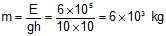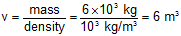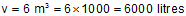Request a call back

the power of a pump motor is 2 kW. how much water can it raise to a height of 10 m in 5 mins?
Asked by Ekta Singh | 09 Sep, 2013, 01:18: PMExpert Answer
The energy of the pump is E = P×t = 2000 × 5 × 60 = 6 × 105 J

Energy is work done to move the water of mass m upto a height h.
E = mgh

Thus, mass of water raised is;Thus, the amount of water raised is equal to the volume of water.Thus, amount of water in litres isAnswered by Romal Bhansali | 10 Sep, 2013, 06:27: PM